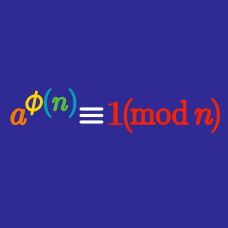Number Theory

# Diffie-Hellman

Solving which of the following problems would allow one to break the Diffie-Hellman protocol?

Suppose Alice and Bob choose $p=191$ and $g=2$. If Alice's secret number is $12$ and Bob's is $16$, what is the shared secret key?

Which of the following primes is most likely to be used in the Diffie-Hellman protocol?

Alice and Bob unwisely choose $p=211$ for their Diffie-Hellman protocol, along with $g=2$. Eve sees the transmission $g^n \pmod p = 155$ and the transmission $g^m \pmod p = 96$. What is the shared secret key $g^{mn} \pmod p$?

Which of the following modifications would NOT increase the security of the Diffie-Hellman protocol?

×